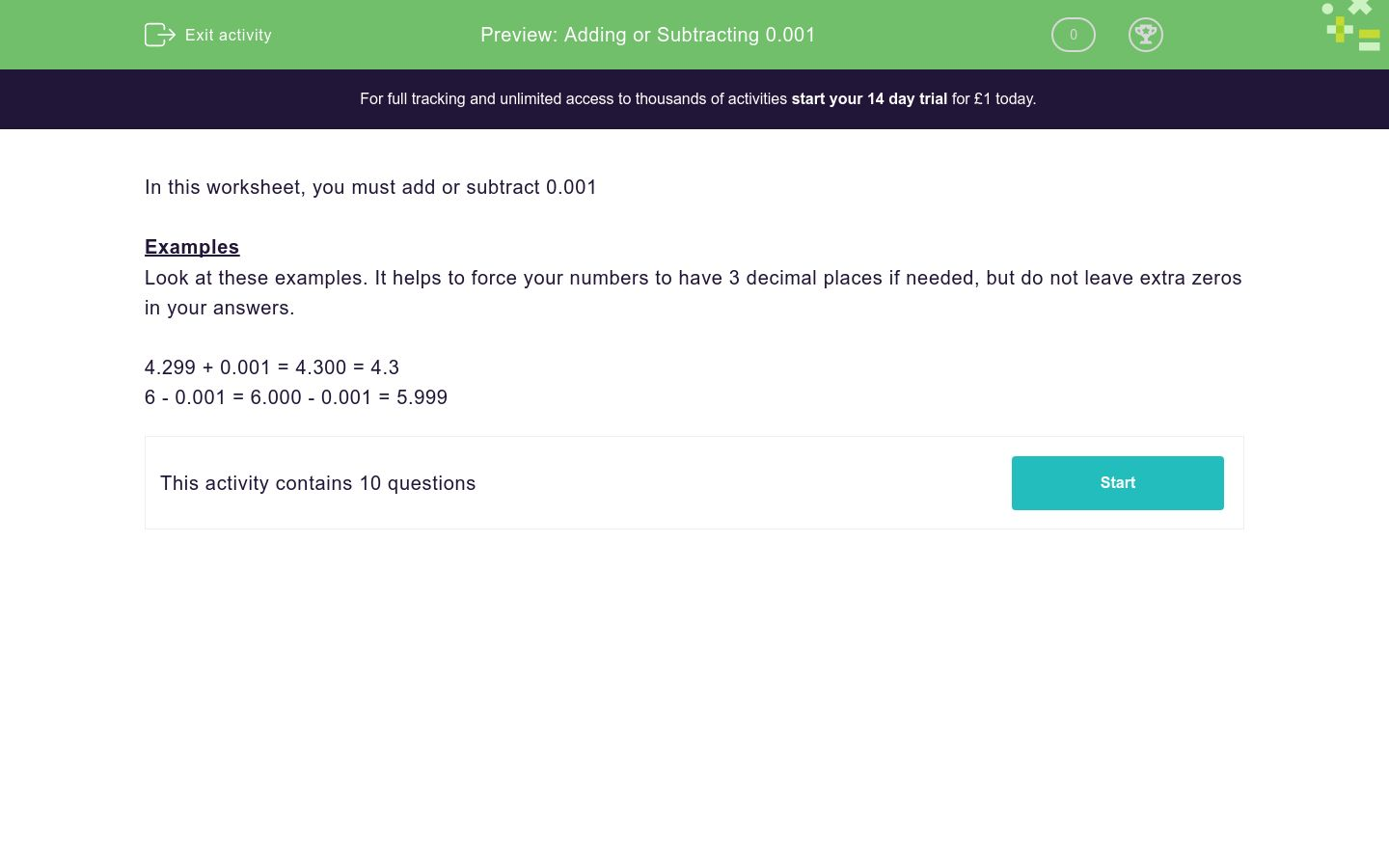### Affordable pricing from £10/month

In this worksheet, students practise adding or subtracting 0.001 to/from numbers.Key stage:  KS 3

Curriculum topic:   Number

Curriculum subtopic:   Use Four Operations for All Numbers

Difficulty level:### QUESTION 1 of 10

In this worksheet, you must add or subtract 0.001

Examples

Look at these examples. It helps to force your numbers to have 3 decimal places if needed, but do not leave extra zeros in your answers.

4.299 + 0.001 = 4.300 = 4.3

6 - 0.001 = 6.000 - 0.001 = 5.999

Work out:

2.643 + 0.001 = __________

Work out:

8.168 + 0.001 = __________

Work out:

3.289 + 0.001 = __________

Work out:

9.299 + 0.001 = __________

Work out:

3.609 + 0.001 = __________

Work out:

5.034 - 0.001 = __________

Work out:

1.657 - 0.001 = __________

Work out:

2.88 - 0.001 = __________

Work out:

4.91 - 0.001 = __________

Work out:

7 - 0.001 = __________

• Question 1

Work out:

2.643 + 0.001 = __________

2.644
• Question 2

Work out:

8.168 + 0.001 = __________

8.169
• Question 3

Work out:

3.289 + 0.001 = __________

3.29
• Question 4

Work out:

9.299 + 0.001 = __________

9.3
• Question 5

Work out:

3.609 + 0.001 = __________

3.61
• Question 6

Work out:

5.034 - 0.001 = __________

5.033
• Question 7

Work out:

1.657 - 0.001 = __________

1.656
• Question 8

Work out:

2.88 - 0.001 = __________

2.879
• Question 9

Work out:

4.91 - 0.001 = __________

4.909
• Question 10

Work out:

7 - 0.001 = __________

6.999
---- OR ----

Sign up for a £1 trial so you can track and measure your child's progress on this activity.

### What is EdPlace?

We're your National Curriculum aligned online education content provider helping each child succeed in English, maths and science from year 1 to GCSE. With an EdPlace account you’ll be able to track and measure progress, helping each child achieve their best. We build confidence and attainment by personalising each child’s learning at a level that suits them.

Get started